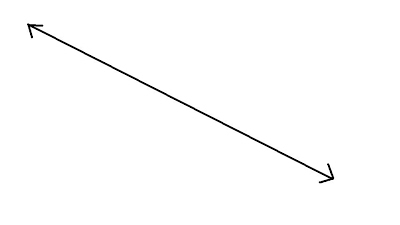100

10 x 58=

580

100What is this called?

A line

100

1/4 + 2/4=

3/4

100

10 x 10=

100

100

What is the answer to a multiplication problem called?

Product

200

900 x 9=

8100

200

A right angle is at what degrees?

90 degrees

200

Change to an improper fraction.

2 1/8

17/8

200

200 x 2 =

400

200

What is the answer to a division problem called?

Quotient

300

13 X 85=

1,105

300
What is the angle called that is less than 90 degree?

Acute

300

Change to a mixed number.

26/3

8 2/3

300

120 + 120 + 20 + 20 + 1=

281

300

Howard arrived to work at the doll head factory at 5:50 a.m. Howard stayed on the factory for 5 hrs. and 10 minutes.  What time did Howard leave the lemonade stand?

11:00 am

400

25 x 64 =

1,600

400What do you call this image?

parallel lines

400

Raf ate 1 1/2 onions before he read his book, and ate another 2 1/2 onions afterward. How many onions did he eat?

4 onions

400

4 times 15

60

400

This triangle has three equal (congruent) sides.

Equilateral Triangle

500

552 x 24=

13,248

500

What is a four sided shape called?

500

5 4/8 + 4 5/8 =

10  1/8

500

4 x 6 + (5 x 4 )

44

500

This triangle has angles that are smaller than 90 degrees.

Acute Triangle

Click to zoom